# Literally equal to (equivLiteral)

## Overview

The Literally equal to (equivLiteral) method checks if the learner response is literally equivalent to the sample correct answer set by the Author, with the exception of some minor syntax differences*. Learner responses that are mathematically equivalent to the authored answer but are written in a different way will be marked as incorrect. By default, this is a strict method but there are additional options, described below, that make the method more flexible in a controlled way. Use the method when you expect the learner to write the answer in a specific format.

*Note that equivLiteral is not performing an exact match as it does not penalize learners for additional whitespace, leading zeros, parentheses which have no specific meaning, choice of multiplication operator, and including a + to denote a positive number by default.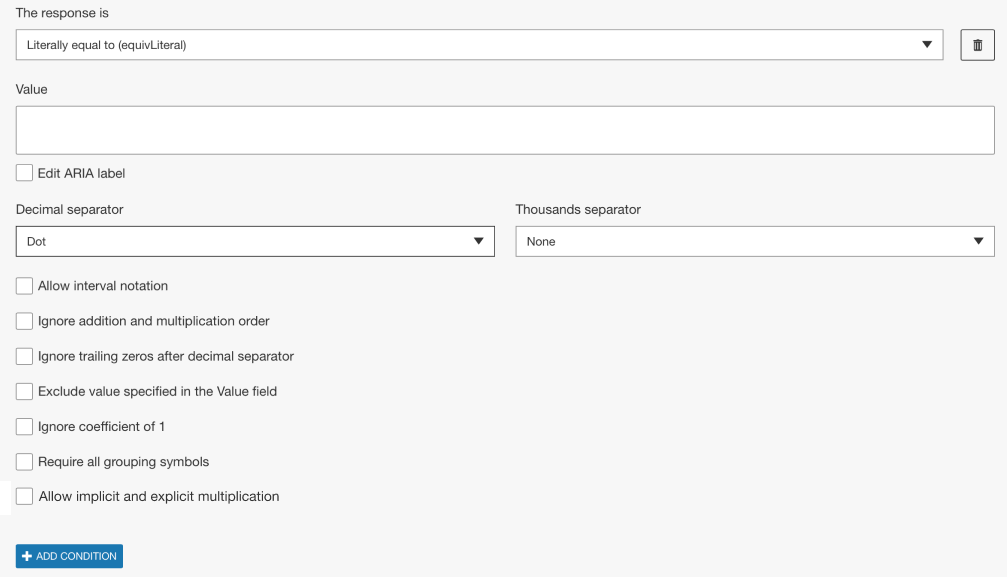Figure 1: Setting the correct answer for equivLiteral in Math Question type.

• Decimal and thousands separator
Authors can specify what separators should be recognized by the engine and can be used by the learner. From the Decimal separator drop-down either a dot or a comma can be selected. Depending on the selection of the decimal separator, from the Thousand Separator drop-down dot, comma, and/or space can be selected.
• Allow interval notation
This option must be enabled when the learner is expected to insert interval notation in the response area, ie `[1, 4)`. Without this option enabled, the response may not validate correctly especially when mis-matched brackets are expected to be used.
• Ignore addition and multiplication order
When enabled the engine will ignore the order in which terms are written with respect to the commutative property of addition and multiplication. For example, if the option is enabled and the authored value is `xy(x+2)`, then `(2+x)yx` would be accepted as a correct learner response.
• Ignore trailing zeros after decimal separator
When enabled the engine will ignore trailing zeros after the decimal separator when comparing the learner response and authored value. For example, if this option is enabled and the authored value is `1000`, then `1000.000` would be accepted as a correct learner response (assuming decimal separator is set as a dot).
• Exclude value specified in Value field
When enabled the value specified by the author in the Value field will not be accepted as a correct answer. This can be a useful way of excluding very specific answers from being marked as correct. Using the option with equivLiteral is often applied as a secondary condition, along with method equivSymbolic, to exclude part of the question being asked.
• Ignore coefficient of 1
When enabled the engine will ignore a coefficient of 1 before a variable when comparing the learner response and authored value. For example, if this option is enable and the authored value is `x+2`, then `1x+2` would be accepted as a correct learner response.
• Require all grouping symbols
By default, without this option enabled, the engine ignores unnecessary parenthesis that do not change the value of the expression. Enable this flag when requiring parenthesis grouping symbols with respect to the associative property of addition and multiplication. For example, if this option is enabled and the authored value is `x+(y+z)`, then `x+y+z` would not be an acceptable answer and would be marked incorrect.

## Examples

### Example 1

In this example we will discuss authoring the following question: Expand the polynomial (x+2)(x+1) and combine like terms. We will set the correct answer as follows and consider how the additional options impact which answers are marked as correct: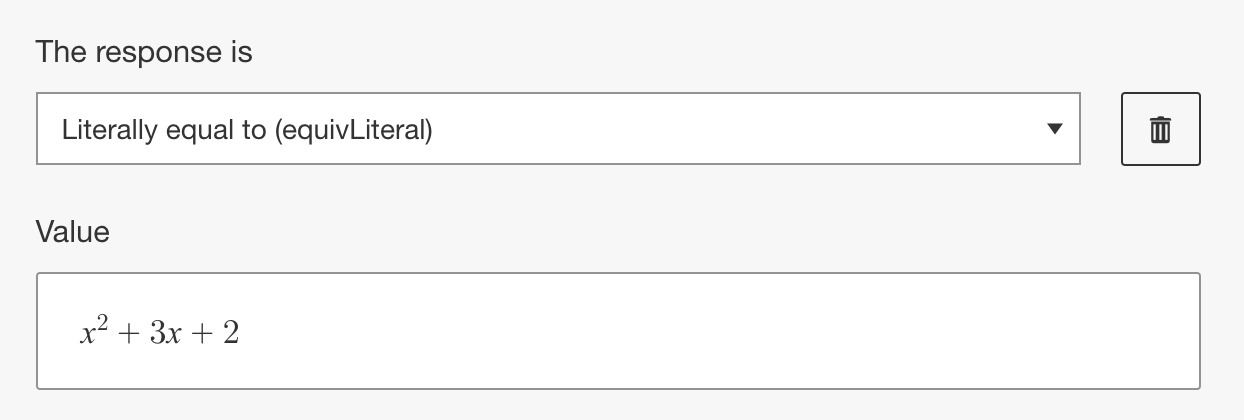If we do not select any of the additional options then we will get the following results:

Learner Response Result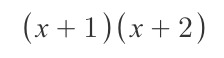False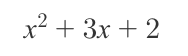True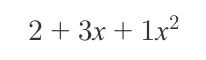False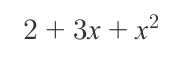False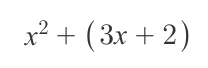True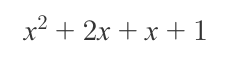False

If we select Ignore addition and multiplication order, then we will get the following results:

Learner Response ResultFalseTrueFalseTrueTrueFalse

If we select Ignore addition and multiplication order and Ignore coefficient of 1, then we will get the following results:

Learner Response ResultFalseTrueTrueTrueTrueFalse

If we select Require all grouping symbols, then we will get the following results:

Learner Response ResultFalseTrueFalseFalseFalseFalse